Question

# HELP!! mastering physics: 7.2: Conservation of Energy with Conservative Forces

Learning Goal: To practice Problem-SolvingStrategy 7.2 Conservation of energy with conservative forces.

A basket of negligible weight hangs from a vertical spring scale offorce constant1500. If you suddenly put an adobebrick of mass3.00in the basket, find the maximum distance thatthespring will stretch.

Problem-Solving Strategy 7.2 Conservation of energy with conservativeforces

SET UP
1. Identify the system youwill analyze, and decide on the initial and final states (positionsand velocities) you will use in solving the problem. Draw oneormore sketches showing the initial and finalstates.
2. Define your coordinatesystem, particularly the zero points for gravitational and elasticpotential energies (the point at whichin the case of gravitational potentialenergy or at which thespring is relaxed in the case of elasticpotential energy).
3. List the initial andfinal kinetic and potential energies?that is,,,, and. Some of these will be known and someunknown. Usealgebraic symbols for any unknown coordinates orvelocities. Keep in mind that potential energyincludes both gravitational and elasticpotential energy.

SOLVE

1. Writeexpressions for the total initial mechanical energy and for thetotal final mechanical energy, equate them, and solve to findwhateverunknown quantity is required.

REFLECT

1. Take a hardlook at your results to see whether they make sense. Are theywithin the general range of magnitudes you expected? If you changeoneof the given quantities, do the results change in a way you canpredict?
SET UPBefore writing any equations, organizeyour information and draw appropriate diagrams.Part AWhich of the following statements about theproblem are true?Check all that apply.

 The spring is initially unstretched. After the brick has been placed in the basket,the spring is stretched. When the brick is first placed into the basket,it has zero velocity. When the brick has stretched the spring to itsfull extent, it has zero velocity. The brick’s final position is lower thanits initial position.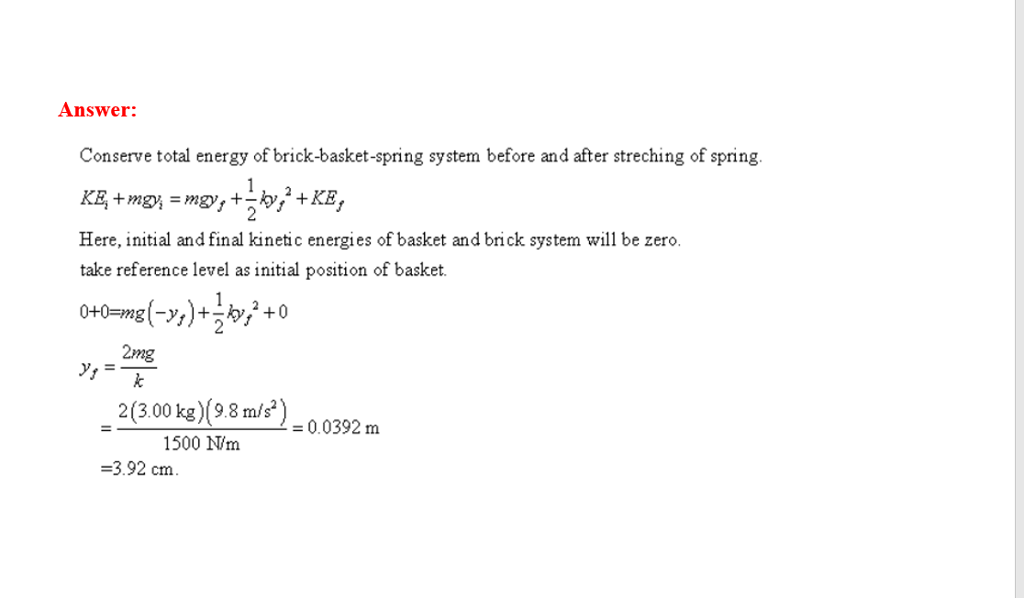They are all true statements.

source: I just did the same assignment

#### Earn Coins

Coins can be redeemed for fabulous gifts.

Similar Homework Help Questions
• ### Conservation of Energy with Conservative Forces

I'm not understanding how conservative and nonconservative forcesinteract. I have a question assigned by my professor:A basket of negligible weight hangs from a vertical spring scale offorce constant1500 . If you suddenly put an adobebrick of mass3.00 in the basket, find the maximum distance thatthespring will stretch.There is a question that states (check all that apply) that aretrue: The spring is initially unstretched.After the brick has been placed in the basket,the spring is stretched.When the brick is first placed into...

• ### conservation of energy multiple choice

Learning Goal: To practice Problem-SolvingStrategy 7.2 Conservation of energy with conservative forces.A basket of negligible weight hangs from a vertical spring scale offorce constant 1500 . If you suddenly put an adobe brick of mass3.00 in the basket, find the maximumdistance that the springwill stretch.Which of the following statements about theproblem are true?The spring is initially unstretched.After the brick has been placed in the basket, the spring isstretched.When the brick is first placed into the basket, it has zerovelocity.When the...

• ### mastering physics: 7.3: The Careless Roofer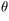Learning Goal: To practice Problem-SolvingStrategy 7.3 Conservation of energy with nonconservativeforces.While a roofer is working on a roof that slants at = 36.0 above the horizontal,heaccidentally nudges his = 8.50 toolbox, causing it to start sliding downward,starting from rest. Africtional force of magnitude = 22.0 acts on the toolbox as it slides. If the boxstarts = 4.25 from the lower edge of the roof, how fast will the toolbox be moving just as itreaches the edge of the roof? Assume...

• ### DECENDING HOOPLearning Goal: To practice Problem-SolvingStrategy 9.1 Rotational energy.A string is attached to the rim of a small hoop of radius = 8.00×10−2 and mass = 0.180 and then wrapped several times around therim. If thefree end of the string is held in place and the hoop is releasedfrom rest and allowed to drop, as shown in the figure (Intro 1figure), calculate the angularspeed and the translational speed of the rotating hoop after it hasdescended = 0.750 . Use = 9.80...

• ### Conservation of energy in charge interactions

To practice Problem-Solving Strategy 21.1 Conservation of energy in charge interactions.An alpha particle (), which is the same as a helium-4 nucleus, is momentarily at rest in a region of space occupied by an electric field. The particle then begins tomove. Find the speed of the alpha particle after it has moved through a potential difference of -3.45×10-3 .The charge and the mass of an alpha particle are = 3.20×10-19 and = 6.68×10-27 , respectively.PROBLEM-SOLVING STRATEGY Conservation of energy in...

• ### PROBLEM 2 (5 points). Momentum and Mechanical Energy conservation A rifle bullet with mass 150 g...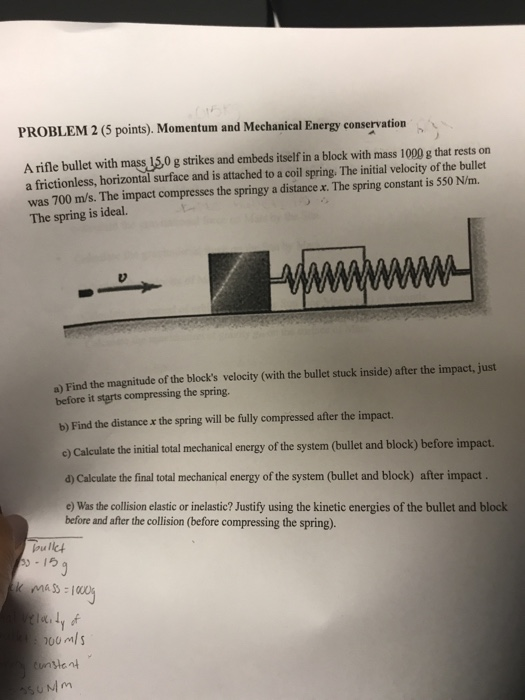PROBLEM 2 (5 points). Momentum and Mechanical Energy conservation A rifle bullet with mass 150 g strikes and embeds itself in a block with mass 1000 g that rests on a frictionless, horizontal surface and is attached to a coil spring. The initial velocity of the bullet was 700 m/s. The impact compresses the springy a distance x. The spring constant is 550 N/m. The spring is ideal. a) Find the magnitude of the block's velocity (with the bullet stuck...

• ### PSS 29.1 Conservation of Energy in Charge Interactions

x.øi5ss="transitionText" style="font-family: Arial, Times, serif; font-size: 10pt; text-align: left; margin-top: 5px; margin-right: 0px; margin-bottom: 10px;margin-left: 0px; line-height: 1.4em; ">PROBLEM-SOLVING STRATEGY 29.1Conservation of energy in charge interactionsMODEL:Check whether there are any dissipative forces that would prevent the mechanical energy from beingconserved.VISUALIZE:Draw a before-and-after pictorial representation. Define symbols that will be used in the problem,list known values, and identify what you are trying to find.SOLVE:The mathematical representation is based on the law of conservation of mechanicalenergy:.Is the electric potential given in the...

• ### NEED HELP with...Energy of Harmonic OscillatorsLearning Goal: To learn to apply the law ofconservation of energy to the analysis of harmonic oscillators.Systems in simple harmonic motion, or harmonicoscillators, obey the law of conservation of energy just likeall other systems do. Using energyconsiderations, one can analyzemany aspects of motion of the oscillator. Such an analysis can besimplified if one assumes that mechanical energy is notdissipated.In other words,,where is the total mechanical energy of the system, is the kinetic energy, and is the potential energy.As you know,...

• ### Application of Universal Gravity non-orbital motion Kinetic Energy mp2 Escape velocity EX-11 Find the Minimum speed...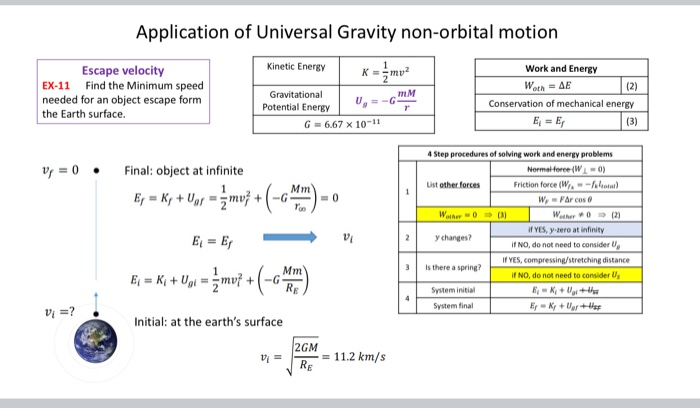Application of Universal Gravity non-orbital motion Kinetic Energy mp2 Escape velocity EX-11 Find the Minimum speed needed for an object escape form the Earth surface. Gravitational mM Potential Energy U = -G G = 6.67 x 10-11 Work and Energy Woth = AE (2) Conservation of mechanical energy E = E (3) V = 0 Final: object at infinite Ey = y + Ug = źmv} + (-GMT) =0 4 Step procedures of solving work and energy problems Normal force...

• ### Conservation of energy In the examples you have examined in this lab, as the coaster travels...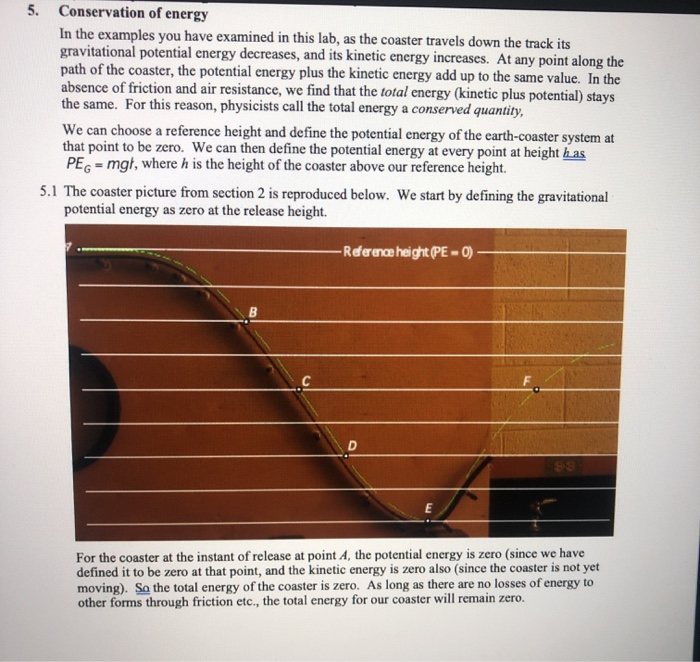Conservation of energy In the examples you have examined in this lab, as the coaster travels down the track its gravitational potential energy decreases, and its kinetic energy increases. At any point along the path of the coaster, the potential energy plus the kinetic energy add up to the same value. In the absence of friction and air resistance, we find that the total energy (kinetic plus potential) stays the same. For this reason, physicists call the total energy a...